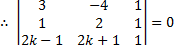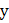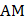# Let, letbe a variable point such thatis the minimum. thenis a) 7/9 b) 0 c) 7/8 d) None of these

## Question ID - 52939 :- Let, letbe a variable point such thatis the minimum. thenis a) 7/9 b) 0 c) 7/8 d) None of these

3537

(c)

We know that(by triangle inequality)

So,is the minimum if, i.e.,are collinearorororNext Question :.andare the variable points of-axis such thatlies belowand. Line joiningandintersect at ''. Locus of '' is a)b)c)d)